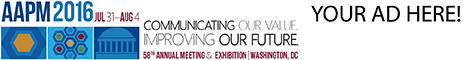# Program Information

## Fast Photon Convolution Calculation with a 3D-Ideal Kernel On the GPUS Moriya

## Presentations

#### SU-E-T-423 (Sunday, July 12, 2015) 3:00 PM - 6:00 PM Room: Exhibit Hall

Purpose: The calculation time is a trade-off for improving the accuracy of convolution dose calculation with fine calculation spacing of the KERMA kernel. We investigated to accelerate the convolution calculation using an ideal kernel on the Graphic Processing Units (GPU).
Methods: The calculation was performed on the AMD graphics hardware of Dual FirePro D700 and our algorithm was implemented using the Aparapi that convert Java bytecode to OpenCL. The process of dose calculation was separated with the TERMA and KERMA steps. The dose deposited at the coordinate (x, y, z) was determined in the process. In the dose calculation running on the central processing unit (CPU) of Intel Xeon E5, the calculation loops were performed for all calculation points. On the GPU computation, all of the calculation processes for the points were sent to the GPU and the multi-thread computation was done. In this study, the dose calculation was performed in a water equivalent homogeneous phantom with 150³ voxels (2 mm calculation grid) and the calculation speed on the GPU to that on the CPU and the accuracy of PDD were compared.
Results: The calculation time for the GPU and the CPU were 3.3 sec and 4.4 hour, respectively. The calculation speed for the GPU was 4800 times faster than that for the CPU. The PDD curve for the GPU was perfectly matched to that for the CPU.
Conclusion: The convolution calculation with the ideal kernel on the GPU was clinically acceptable for time and may be more accurate in an inhomogeneous region. Intensity modulated arc therapy needs dose calculations for different gantry angles at many control points. Thus, it would be more practical that the kernel uses a coarse spacing technique if the calculation is faster while keeping the similar accuracy to a current treatment planning system.

Contact Email: